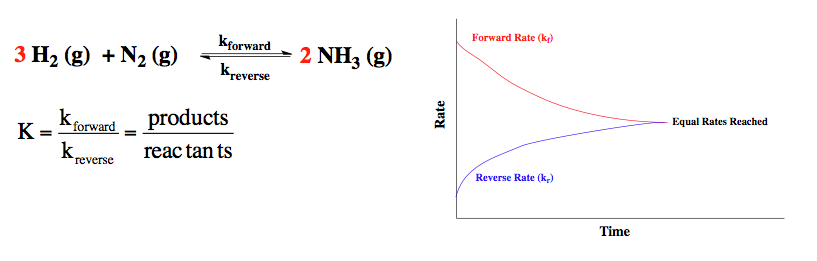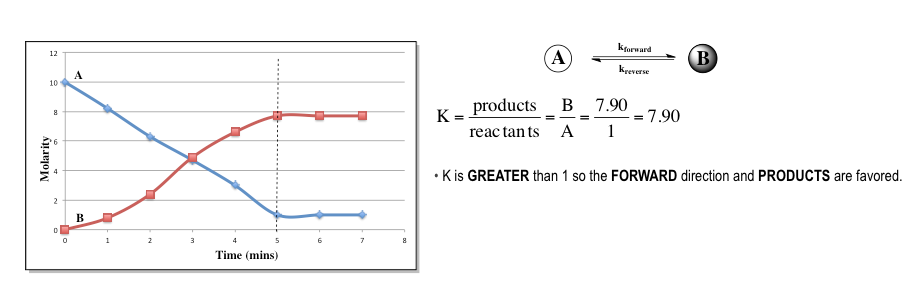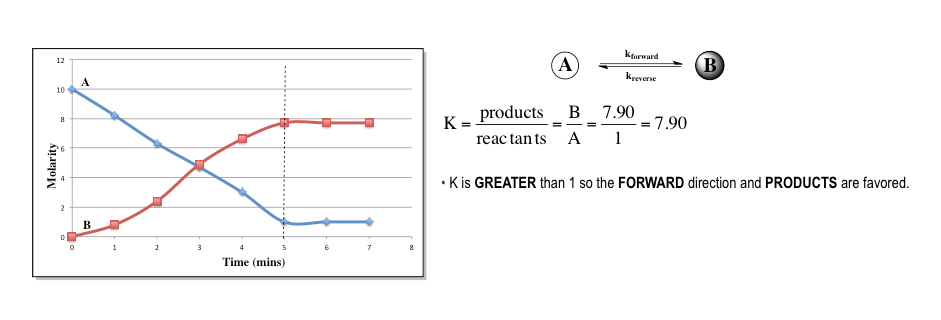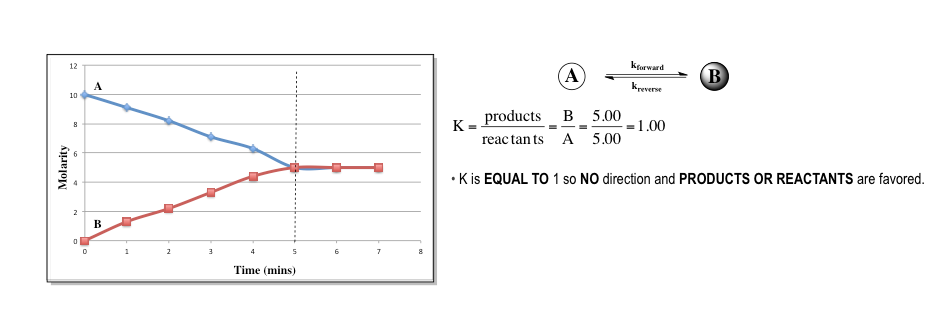Clutch Prep is now a part of Pearson
Ch.6 - Chemical EquilibriumWorksheetSee all chapters

# The Equilibrium State

See all sections
Sections
The Equilibrium State
The Reaction Quotient
Le Chatelier's Principle
Chemical Thermodynamics: Enthalpy
Chemical Thermodynamics: Entropy
Chemical Thermodynamics: Gibbs Free Energy
Solubilty Product Constant
Protic Acids and Bases
The pH Scale
Acid Strength

Under an equilibrium state the rates of the reverse and forward reaction are equal.

###### The Equilibrium State

Concept #1: Most chemical reactions do not go to completion and instead reach a state of chemical equilibrium.

Equilibrium is reached when the rates of the forward and reverse reaction are equal.The magnitude of K determines which direction is favored at equilibrium. If K is greater than 1 then products are favored over reactants.If K is less than 1 then reactants are favored over products.If K is equal to 1 then both reactants and products are favored.###### Equilibrium State Calculations

Example #1: For the following chemical reaction N2 (g) + O2 (g)  2 NO (g), Kc = 3.7 x 10-5, kf = 2.5 x 10-3 and kr = 67.57. Addition of a catalyst increases the forward rate constant 1.8 x 10-1. What is the new reverse rate constant after the addition of the catalyst?

Example #2: Consider the following reactions at 25°C:

Reaction                                                                      Kc
2 NO (g) ⇌ N(g) + O(g)                             1 × 1030
2 H2O (g) ⇌ 2 H(g) + O(g)                       5 × 10−82
2 CO (g) + O(g) ⇌ 2 CO(g)                      3 × 1091

Which compound is most likely to dissociate and give O(g) at 25°C?

a) CO2                          b) NO                           c) CO                           d) H2O

Practice: Write the equilibrium expression for the following reaction.

4 NH(g) + 3 O(g) ⇌  2 N(g) + 6 H2O (l)

Example #3: When reaction 1 and 2 below are added together, the result is reaction 3.

1)  H2O (l) + HNO(aq) ⇌ H3O+ (aq) + NO2 (aq)              K1 = 4.50 × 104

2)  H3O+ (aq) + OH (aq) ⇌ 2 H2O (l)                                        K2 = 1.00 × 1014

3)  HNO(aq) + OH (aq) ⇌ NO2 (aq) + H2O (l)                 K= ?

Find the equilibrium constant, K3.

A) 4.50 × 1018        B) 2.22 × 1017        C) 4.50 × 1010        D) 1.00 × 1014

Example #4: What is the equilibrium constant for the reaction

NH3 (aq) + H3O+ (aq) ⇌ NH4+ (aq) + H2O (l)

Given the following information:

NH3 (aq) + H2O (l)  ⇌   NH4+ (aq) + OH (aq)  Kc = 1.8 × 10‒5

4 H2O (l) ⇌ 2 OH (aq)  + 2 H3O+ (aq)  Kc = 1 × 10‒28

a) 1.8 × 109        b) 4.5 × 107        c) 9.0 × 1010        d) 1.8 × 10-5           e) 2.7 × 106